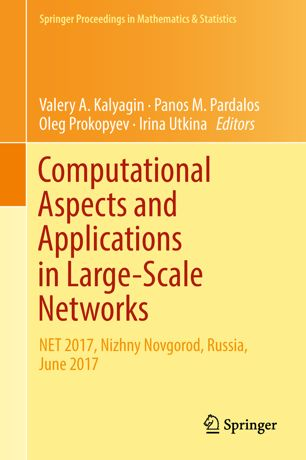• A
• A
• A
• ABC
• ABC
• ABC
• А
• А
• А
• А
• А
Regular version of the site

## FPT Algorithms for the Shortest Lattice Vector and Integer Linear Programming Problems

P. 19-35.

In this paper, we present FPT algorithms for special cases of the shortest vector problem (SVP) and the integer linear programming problem (ILP), when matrices included in the problems’ formulations are near square. The main parameter is the maximal absolute value of rank minors of matrices included in the problem formulation. Additionally, we present FPT algorithms with respect to the same main parameter for the problems, when the matrices have no singular rank sub-matrices.

### In bookValery A. Kalyagin, Panos M. Pardalos, Oleg Prokopyev et al. Vol. 247. Springer, 2018.